Week 2-C Power Series

Friday, May 17, 2013

2:13 PM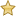Formal Power Series

A formal power series is a power series where we don't care about convergence.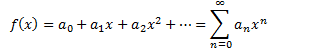Notation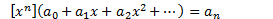•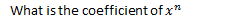•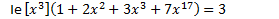Formal power series can be added and multiplied in the obvious way.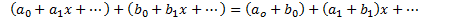Equivalently,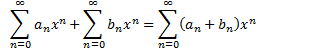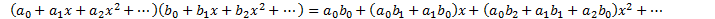Equivalently,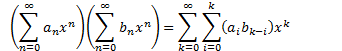Example

Let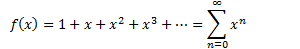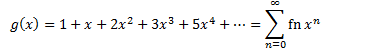• Fn = Fibonacci number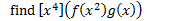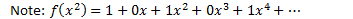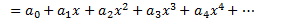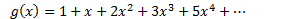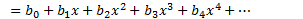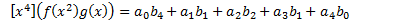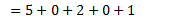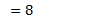Example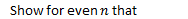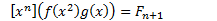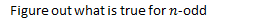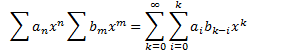For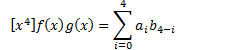Multiplicative inverse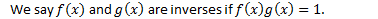Example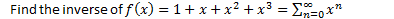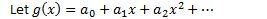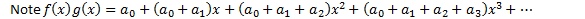Note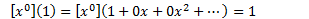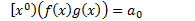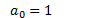Note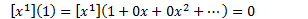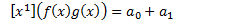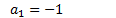Note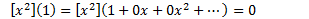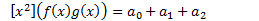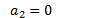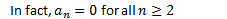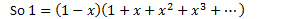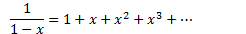Example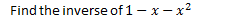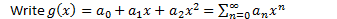We want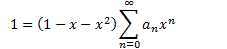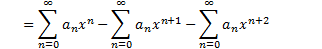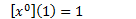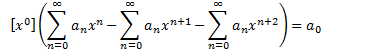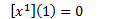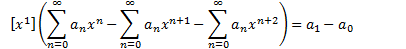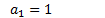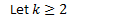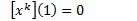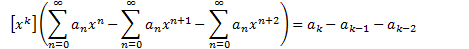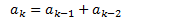These are the Fibonacci numbers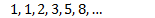Created with Microsoft OneNote 2010
One place for all your notes and information